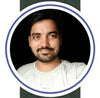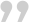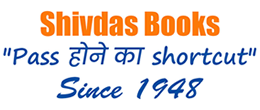## TRENDING BOOKS

Rs. 105.00Rs. 150.00

Rs. 105.00Rs. 150.00

Rs. 105.00Rs. 150.00

Rs. 350.00Rs. 500.00

Rs. 350.00Rs. 500.00

Rs. 298.00Rs. 425.00

Rs. 210.00Rs. 300.00

Rs. 252.00Rs. 360.00

Rs. 252.00Rs. 360.00

Rs. 210.00Rs. 300.00

Rs. 210.00Rs. 300.00

Rs. 210.00Rs. 300.00

Rs. 210.00Rs. 300.00

Rs. 210.00Rs. 300.00

Rs. 210.00Rs. 300.00

Rs. 210.00Rs. 300.00

Rs. 210.00Rs. 300.00

Rs. 350.00Rs. 500.00

Rs. 840.00Rs. 1,200.00

Rs. 210.00Rs. 300.00

Rs. 210.00Rs. 300.00

Rs. 210.00Rs. 300.00

Rs. 392.00Rs. 560.00

Rs. 350.00Rs. 500.00

Rs. 392.00Rs. 560.00

Rs. 1,330.00Rs. 1,900.00

Rs. 455.00Rs. 650.00

Rs. 420.00Rs. 600.00

Rs. 105.00Rs. 150.00

Rs. 105.00Rs. 150.00

Rs. 105.00Rs. 150.00

Rs. 350.00Rs. 500.00

Rs. 350.00Rs. 500.00

Rs. 298.00Rs. 425.00

Rs. 252.00Rs. 360.00

Rs. 252.00Rs. 360.00

Rs. 210.00Rs. 300.00

Rs. 210.00Rs. 300.00

Rs. 210.00Rs. 300.00

Rs. 252.00Rs. 360.00

Rs. 420.00Rs. 600.00

Rs. 315.00Rs. 450.00

Rs. 750.00Rs. 1,250.00

Rs. 175.00Rs. 250.00

Rs. 175.00Rs. 250.00

Rs. 175.00Rs. 250.00

Rs. 175.00Rs. 250.00

Rs. 175.00Rs. 250.00For the Past 6 Years, I am using the past the 7 years and Chapterwise books of the Shiv Das Publication for teaching my students.
It is the best prepration book for CBSE Board Exams and also it heps to score high marks.Praveen Maths PGTWith my personal experience, I would say Shiv Das Books are the best choice for CBSE Books. If you're a student of class 12 you should prepare their sample papers. You should check previous year papers too. Almost 90% questions you will find have come from this book.Sachin Kumar
Oriental Classes, DelhiHappy Students

Successful Years

Teachers Associated

Bookshop Affiliated